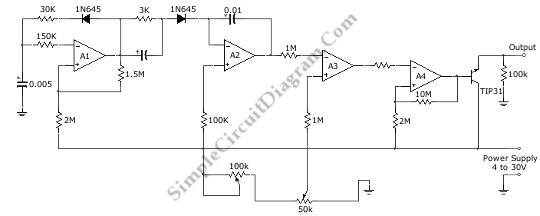# Pulse Width (PWM) Controller Circuit

A LM3900 quad operational amplifier can be used to build a pulse width (PWM) controller. The LM3900 was chosen because it only need single supply voltage of 4 to 30 V. This circuit can give 100 percent pulse-width control.  A1 op-amp forms a 1 kHz oscillator, generating a square wave oscillator for the basic oscillation. A2 is a ramp generator which convert the square wave to a sawtooth wave,  producing a linear ramp voltage for each oscillator pulse. Then this ramp signal is fed to inverting input of comparator A3, to produce a repetitive pulse which has variable width which is proportional to the control voltage applied to the noninverting input. Here is the schematic diagram of the circuit: